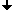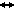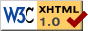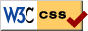Nonlinear Dynamics at the Free University Berlin

DFG Priority Research Program DANSE on
Ergodic theory, analysis, and efficient simulation of dynamical systems

Research Project

# Exponentially small effects in parabolic PDE

Research director
Prof. Dr. Bernold Fiedler
Members
Dr. Karsten Matthies

## Summary

We analyze exponentially small effects in parabolic partial differential equations. These effects are encountered in ordinary differential equations when the equation is discretized with step-size h or perturbed by non-autonomous h-periodic forcing. Important results about the exponentially small difference between continuous flows and iterations were given by Neishtadt, Lazutkin and inside the research program by Lubich, Scheurle and Fiedler.

Similar results for partial differential equations are missing up to now completely and are the aim of the project. Using results on exponentially dichotomies it is possible to show the exponentially smallness of the splitting of homoclinic orbits in the non-autonomous parabolic case. Semidiscretization in time does not directly correspond to time-periodic forcing, as it does in the case of ordinary differential equations. Therefore we consider as an example full discretization by a Galerkin method in space with Gevrey regularity and some Runge-Kutta discretization in time.Last change: Dec. 6, 2003
 This page strictly conforms to the XHTML1.0 standard and uses style sheets.